# seaborn.JointGrid#

class seaborn.JointGrid(data=None, *, x=None, y=None, hue=None, height=6, ratio=5, space=0.2, palette=None, hue_order=None, hue_norm=None, dropna=False, xlim=None, ylim=None, marginal_ticks=False)#

Grid for drawing a bivariate plot with marginal univariate plots.

Many plots can be drawn by using the figure-level interface `jointplot()`. Use this class directly when you need more flexibility.

__init__(data=None, *, x=None, y=None, hue=None, height=6, ratio=5, space=0.2, palette=None, hue_order=None, hue_norm=None, dropna=False, xlim=None, ylim=None, marginal_ticks=False)#

Set up the grid of subplots and store data internally for easy plotting.

Parameters:
data`pandas.DataFrame`, `numpy.ndarray`, mapping, or sequence

Input data structure. Either a long-form collection of vectors that can be assigned to named variables or a wide-form dataset that will be internally reshaped.

x, yvectors or keys in `data`

Variables that specify positions on the x and y axes.

heightnumber

Size of each side of the figure in inches (it will be square).

rationumber

Ratio of joint axes height to marginal axes height.

spacenumber

Space between the joint and marginal axes

dropnabool

If True, remove missing observations before plotting.

{x, y}limpairs of numbers

Set axis limits to these values before plotting.

marginal_ticksbool

If False, suppress ticks on the count/density axis of the marginal plots.

huevector or key in `data`

Semantic variable that is mapped to determine the color of plot elements. Note: unlike in `FacetGrid` or `PairGrid`, the axes-level functions must support `hue` to use it in `JointGrid`.

palettestring, list, dict, or `matplotlib.colors.Colormap`

Method for choosing the colors to use when mapping the `hue` semantic. String values are passed to `color_palette()`. List or dict values imply categorical mapping, while a colormap object implies numeric mapping.

hue_ordervector of strings

Specify the order of processing and plotting for categorical levels of the `hue` semantic.

hue_norm

Either a pair of values that set the normalization range in data units or an object that will map from data units into a [0, 1] interval. Usage implies numeric mapping.

`jointplot`

Draw a bivariate plot with univariate marginal distributions.

`PairGrid`

Set up a figure with joint and marginal views on multiple variables.

`jointplot`

Draw multiple bivariate plots with univariate marginal distributions.

Examples

Calling the constructor initializes the figure, but it does not plot anything:

```penguins = sns.load_dataset("penguins")
sns.JointGrid(data=penguins, x="bill_length_mm", y="bill_depth_mm")
```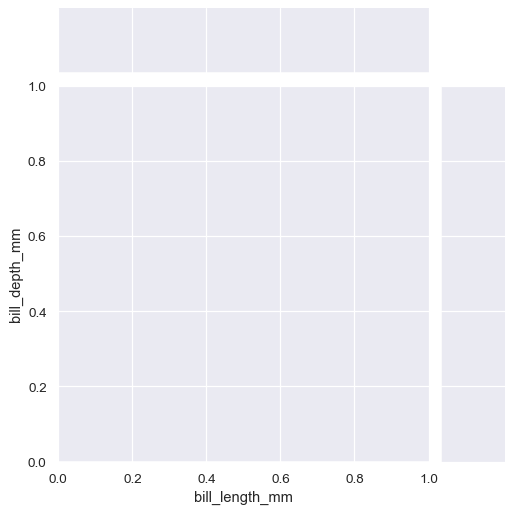The simplest plotting method, `JointGrid.plot()` accepts a pair of functions (one for the joint axes and one for both marginal axes):

```g = sns.JointGrid(data=penguins, x="bill_length_mm", y="bill_depth_mm")
g.plot(sns.scatterplot, sns.histplot)
```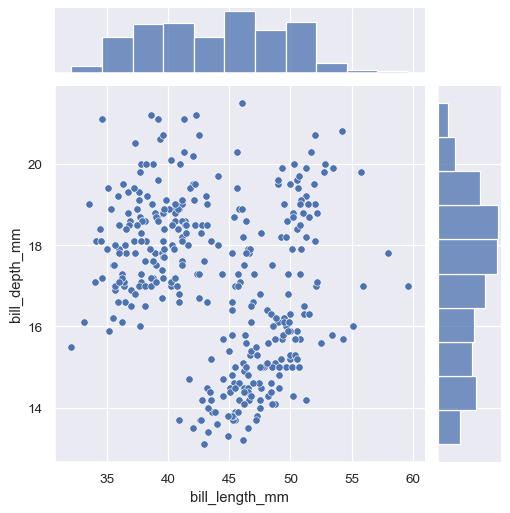The `JointGrid.plot()` function also accepts additional keyword arguments, but it passes them to both functions:

```g = sns.JointGrid(data=penguins, x="bill_length_mm", y="bill_depth_mm")
g.plot(sns.scatterplot, sns.histplot, alpha=.7, edgecolor=".2", linewidth=.5)
```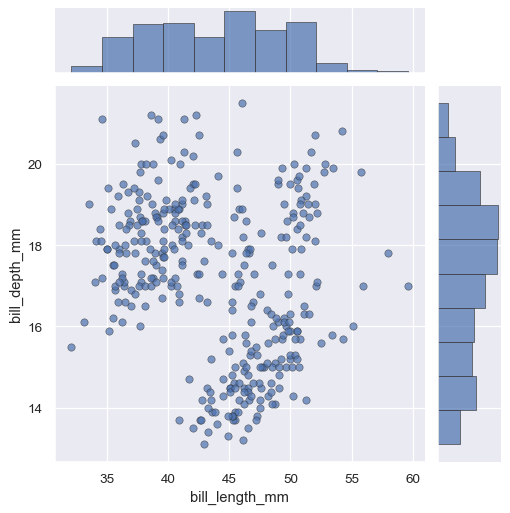If you need to pass different keyword arguments to each function, you’ll have to invoke `JointGrid.plot_joint()` and `JointGrid.plot_marginals()`:

```g = sns.JointGrid(data=penguins, x="bill_length_mm", y="bill_depth_mm")
g.plot_joint(sns.scatterplot, s=100, alpha=.5)
g.plot_marginals(sns.histplot, kde=True)
```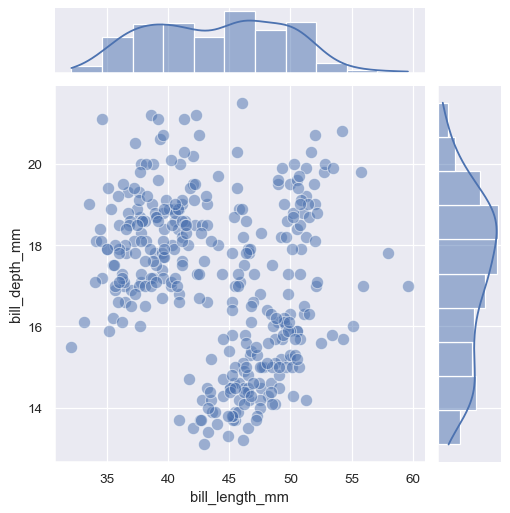You can also set up the grid without assigning any data:

```g = sns.JointGrid()
```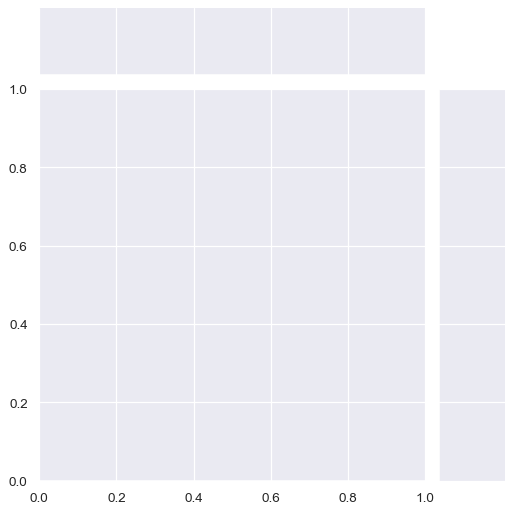You can then plot by accessing the `ax_joint`, `ax_marg_x`, and `ax_marg_y` attributes, which are `matplotlib.axes.Axes` objects:

```g = sns.JointGrid()
x, y = penguins["bill_length_mm"], penguins["bill_depth_mm"]
sns.scatterplot(x=x, y=y, ec="b", fc="none", s=100, linewidth=1.5, ax=g.ax_joint)
sns.histplot(x=x, fill=False, linewidth=2, ax=g.ax_marg_x)
sns.kdeplot(y=y, linewidth=2, ax=g.ax_marg_y)
```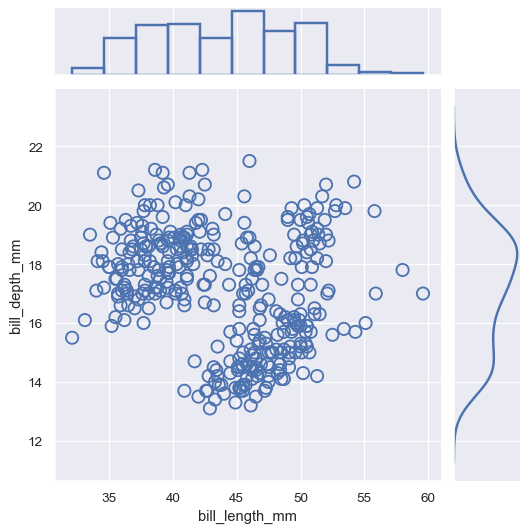The plotting methods can use any seaborn functions that accept `x` and `y` variables:

```g = sns.JointGrid(data=penguins, x="bill_length_mm", y="bill_depth_mm")
g.plot(sns.regplot, sns.boxplot)
```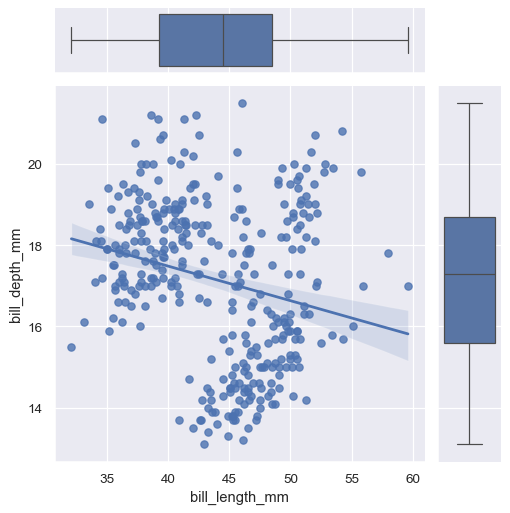If the functions accept a `hue` variable, you can use it by assigning `hue` when you call the constructor:

```g = sns.JointGrid(data=penguins, x="bill_length_mm", y="bill_depth_mm", hue="species")
g.plot(sns.scatterplot, sns.histplot)
```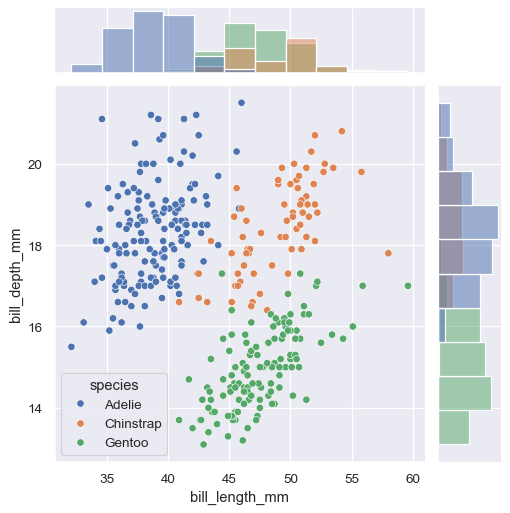Horizontal and/or vertical reference lines can be added to the joint and/or marginal axes using :meth:`JointGrid.refline`:

```g = sns.JointGrid(data=penguins, x="bill_length_mm", y="bill_depth_mm")
g.plot(sns.scatterplot, sns.histplot)
g.refline(x=45, y=16)
```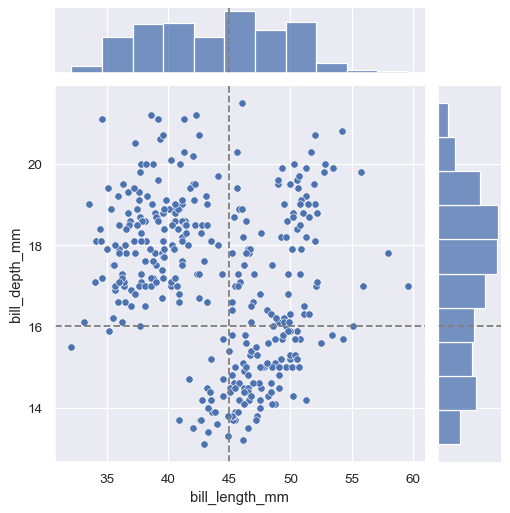The figure will always be square (unless you resize it at the matplotlib layer), but its overall size and layout are configurable. The size is controlled by the `height` parameter. The relative ratio between the joint and marginal axes is controlled by `ratio`, and the amount of space between the plots is controlled by `space`:

```sns.JointGrid(height=4, ratio=2, space=.05)
```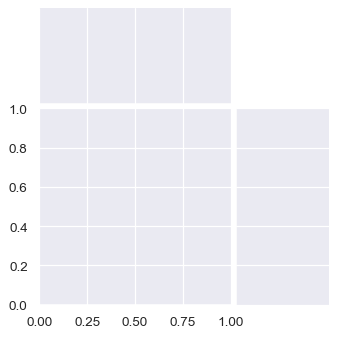By default, the ticks on the density axis of the marginal plots are turned off, but this is configurable:

```sns.JointGrid(marginal_ticks=True)
```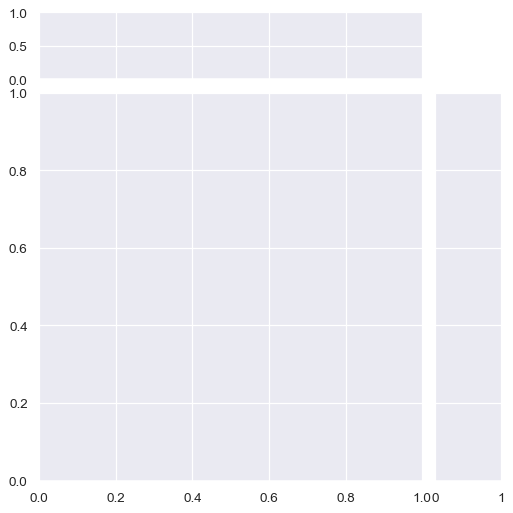Limits on the two data axes (which are shared across plots) can also be defined when setting up the figure:

```sns.JointGrid(xlim=(-2, 5), ylim=(0, 10))
```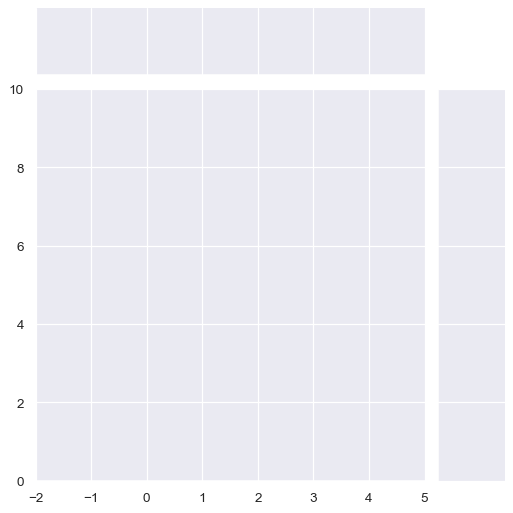Methods

 `__init__`([data, x, y, hue, height, ratio, ...]) Set up the grid of subplots and store data internally for easy plotting. `apply`(func, *args, **kwargs) Pass the grid to a user-supplied function and return self. `pipe`(func, *args, **kwargs) Pass the grid to a user-supplied function and return its value. `plot`(joint_func, marginal_func, **kwargs) Draw the plot by passing functions for joint and marginal axes. `plot_joint`(func, **kwargs) Draw a bivariate plot on the joint axes of the grid. `plot_marginals`(func, **kwargs) Draw univariate plots on each marginal axes. `refline`(*[, x, y, joint, marginal, color, ...]) Add a reference line(s) to joint and/or marginal axes. `savefig`(*args, **kwargs) Save an image of the plot. `set`(**kwargs) Set attributes on each subplot Axes. `set_axis_labels`([xlabel, ylabel]) Set axis labels on the bivariate axes.

Attributes

 `fig` DEPRECATED: prefer the `figure` property. `figure` Access the `matplotlib.figure.Figure` object underlying the grid.